# Newton’s Laws of MotionPage 2

#### WATCH ALL SLIDES

In the absence of a force of friction, the book would continue in motion with the same speed and direction - forever! (Or at least to the end of the table top.)

Slide 12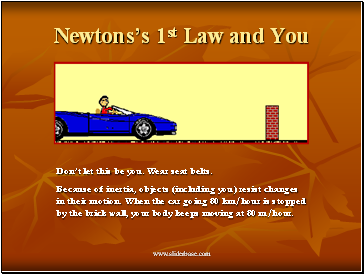Newtons’s 1st Law and You

Don’t let this be you. Wear seat belts.

Because of inertia, objects (including you) resist changes in their motion. When the car going 80 km/hour is stopped by the brick wall, your body keeps moving at 80 m/hour.

Slide 132nd Law

F = m x a

Slide 14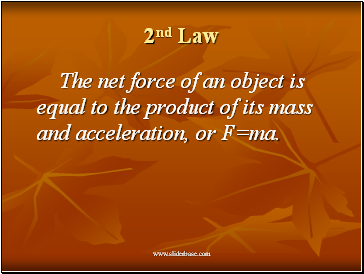2nd Law

The net force of an object is equal to the product of its mass and acceleration, or F=ma.

Slide 152nd Law

When mass is in kilograms and acceleration is in m/s/s, the unit of force is in newtons (N).

One newton is equal to the force required to accelerate one kilogram of mass at one meter/second/second.

Slide 16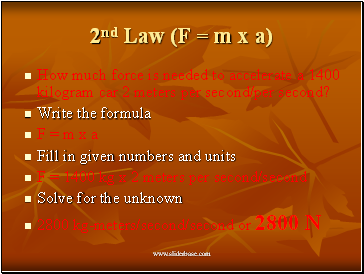2nd Law (F = m x a)

How much force is needed to accelerate a 1400 kilogram car 2 meters per second/per second?

Write the formula

F = m x a

Fill in given numbers and units

F = 1400 kg x 2 meters per second/second

Solve for the unknown

2800 kg-meters/second/second or 2800 N

Slide 17If mass remains constant, doubling the acceleration, doubles the force. If force remains constant, doubling the mass, halves the acceleration.

Slide 18Newton’s 2nd Law proves that different masses accelerate to the earth at the same rate, but with different forces.

We know that objects with different masses accelerate to the ground at the same rate.

However, because of the 2nd Law we know that they don’t hit the ground with the same force.

F = ma

98 N = 10 kg x 9.8 m/s/s

F = ma

9.8 N = 1 kg x 9.8 m/s/s

Slide 19Slide 20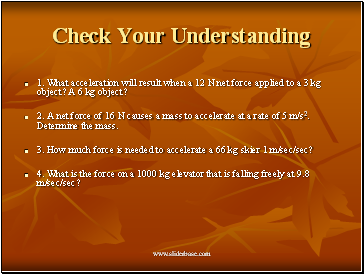1. What acceleration will result when a 12 N net force applied to a 3 kg object? A 6 kg object?

2. A net force of 16 N causes a mass to accelerate at a rate of 5 m/s2. Determine the mass.

3. How much force is needed to accelerate a 66 kg skier 1 m/sec/sec?

4. What is the force on a 1000 kg elevator that is falling freely at 9.8 m/sec/sec?

Slide 21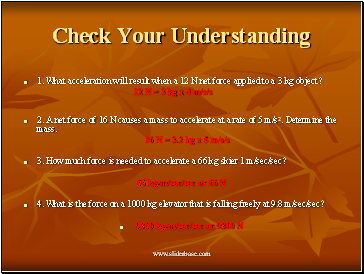Go to page:
1  2  3  4